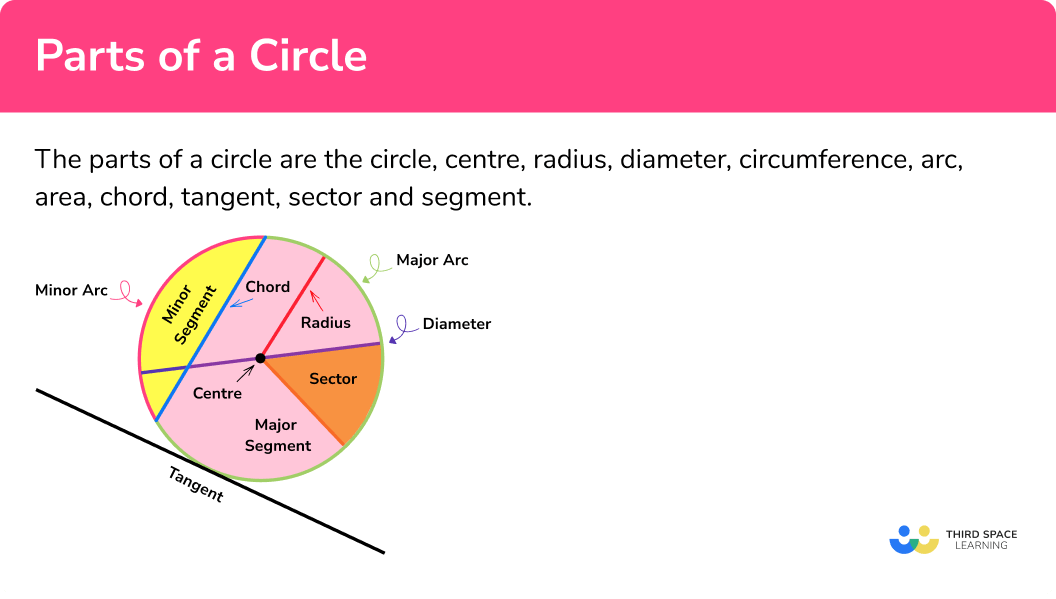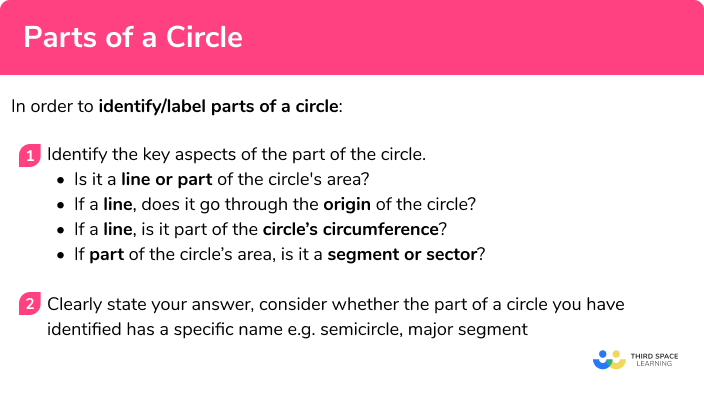# Parts Of A Circle

Here we will learn about the different parts of a circle including how to identify the key parts of a circle from a diagram, how to identify the key parts of a circle from a definition and how to draw a circle with the different parts labelled e.g. the diameter of a circle.

There are also parts of a circle worksheets based on Edexcel, AQA and OCR exam questions, along with further guidance on where to go next if you’re still stuck.

## What are the parts of a circle?

The parts of a circle are the radius, diameter, circumference, arc, chord, secant, tangent, sector and segment.

Circle terms

Naming circle parts:

Circle

A round plane figure whose boundary consists of points equidistant from a fixed point.

Centre

The centre of the circle is the fixed point from which all points on the boundary of the circle are equidistant.

Often noted on diagrams as ‘O’.

Centre/center are the same. Centre is the UK spelling whilst Center is the US spelling.

The distance from the centre of a circle to the outside.

The radius of the circle is half the diameter of the circle.

Diameter

The distance across the circle going through the centre.

The diameter is twice the radius.

Circumference

The distance once around the circle.

Arc

A part of the circumference.

Major arc – A major arc is greater than half the circumference.

Minor arc – A minor arc is less than half the circumference.

Area

The space inside a 2D shape.

Chord

A line segment going from one point of the circumference to another but does not go through the centre.

Secant

A line that goes through the circle at two points.

Note: Secant is not a term you are required to know at GCSE, however it is important to note the difference between a chord and a secant.

Tangent

A straight line that touches the circle at a single point only.

Sector

A section of the circle created by two radii.

Major sector – A major sector has a central angle which is more than 180^o .

Minor sector – A minor sector has a central angle which is less than 180^o .

Semi circle

Half of a circle. Could be considered a sector where the circle has be split by the diameter.

A quarter of a circle, created by two perpendicular radii.

Segment

A section of the circle created by a chord.

Major segment – a segment where the arc is greater than half the circumference.

Minor segment – a segment where the arc is less than half the circumference.

### What are the parts of a circle?Other keys terms:

Equidistance

A point is equidistant from 2 other points when it is always the same distance away from those points.

Angles around a point

Angles around a point are equal to 360^o .

## How to identify parts of a circle

In order to identify/label parts of a circle:

1 Identify the key aspects of the part of the circle.

• Is it a line or part of the circle’s area?
• If a line goes through the origin of the circle?
• If a line, is it part of the circle’s circumference?
• If part of the circle’s area is it a segment or sector?

2 Clearly state your answer, consider whether the part of a circle you have identified has a specific name e.g. semicircle, major segment.

### Explain how to identify parts of a circleParts of a circle is part of our series of lessons to support revision on circles, sectors and arcs. You may find it helpful to start with the main circles, sectors and arcs lesson for a summary of what to expect, or use the step by step guides below for further detail on individual topics. Other lessons in this series include:

## Parts of a circle examples

### Example 1: identifying a part of a circle from a diagram

Name the part of the circle shown in red in the below diagram.

1.  Identify the key aspects of the part of the circle. Here are some key questions you can ask yourself?

• Is it a line or part of the circle’s area?                          Line
• If a line goes through the origin of the circle?             Yes
• If a line, is it part of the circle’s circumference?            No

2Clearly state your answer, consider whether the part of a circle you have identified has a specific name e.g. major segment.

### Example 2: identifying a part of a circle from a diagram

Name the part of the circle shown in red in the below diagram.

Identify the key aspects of the part of the circle. Here are some key questions you can ask yourself?

Clearly state your answer, consider whether the part of a circle you have identified has a specific name e.g. major segment.

### Example 3: identifying a part of a circle from a diagram

Identify the key aspects of the part of the circle. Here are some key questions you can ask yourself?

Clearly state your answer, consider whether the part of a circle you have identified has a specific name e.g. major segment.

### Example 4: identifying a part of a circle from a diagram

Name the part of the circle shown in red in the below diagram.

Identify the key aspects of the part of the circle. Here are some key questions you can ask yourself?

Clearly state your answer, consider whether the part of a circle you have identified has a specific name e.g. major segment.

### Example 5: identifying a part of a circle from a diagram

Identify the key aspects of the part of the circle. Here are some key questions you can ask yourself?

Clearly state your answer, consider whether the part of a circle you have identified has a specific name e.g. major segment.

### Example 6: labelling a part of a circle on a diagram

On the circle below:

Draw a diameter.

Draw a tangent.

Label the circumference.

Identify the key aspects of the part of the circle.

### Common misconceptions

The radius is from the centre of the circle to the circumference whilst the diameter goes across the whole circle whilst going through the origin.

• Confusing the Segment/Sector

A segment is made from a chord whilst a sector will have lines (radii) coming from the origin.

HINT: Some students like to consider a sector like a slice of pizza.

• Confusing the Chord/Diameter

A chord will not go through the origin of the circle whilst the diameter will.

• Confusing the Tangent/Secant

A tangent only touches the circumference at a single point, it does not cross the line. A secant will cross the circumference twice.

### Practice parts of a circle questions

1. If a circle has an ‘o’ noted on it. It normally notes the

Circle is an o shapeMiddle of the circle (origin)Name of the circle is OA circle inside a circleThe ‘o’ refers to the centre of the circle which is called the origin of the circle

2. Which will be the longest in length of any circleDiameterChordCircumferenceThe circumference is the distance around the edge of the circle, this will always be longer

3. Half a circle is called:

A Semi CircleA Half circleA 180 degree circle4. A line that touches the circumference of a circle at one point is called a:

A secantA segmentA TangentAn Arc5. Which of the following statements is true?

The radius is twice the length of the diameterThe diameter is twice the length of the radiusThe radius is half the length of the circumferenceThe diameter is half the length of the circumferenceThe distance from the centre of the circle to the circumference is called the radius. The distance across the circle passing through the centre is called diameter. This means that the diameter is twice as long as the radius.

6. A sector is:

Part of a circle’s circumferencePart of a circle’s areaA line that goes through a circleThe middle of a circleA sector consists of the area created by an arc and two radii

### Parts of a circle GCSE questions

1. Name the part of the circle shown in the diagram below:(1 mark)

Chord

(1)

2. Name the part of the circle shown in the diagram below:(1 mark)

Tangent

(1)

3. Name the part of the circle shown in the diagram below:(1 mark)

Sector

(1)

4. Name the part of the circle shown in the diagram below:(1 mark)

Diameter

(1)

5. Name the part of the circle shown in the diagram below:(1 mark)

(1)

6. Name the part of the circle shown in the diagram below:

‘A line that goes across the circle but does not go through the origin’

(1 mark)

Chord

(1)

7. Molly says ‘A chord is the same as a radius but shorter’. Is Molly correct? Explain your answer

(1 mark)

No, the radius goes from the origin to the circumference. A chord does not touch the origin of the circle

(1)

## Learning checklist

You have now learned how to:

• Identify and apply circle definitions and properties, including: centre, radius, chord, diameter, circumference (F)
• Identify and apply circle definitions and properties, including tangent, arc, sector and segment (F+)

## Still stuck?

Prepare your KS4 students for maths GCSEs success with Third Space Learning. Weekly online one to one GCSE maths revision lessons delivered by expert maths tutors.

Find out more about our GCSE maths tuition programme.